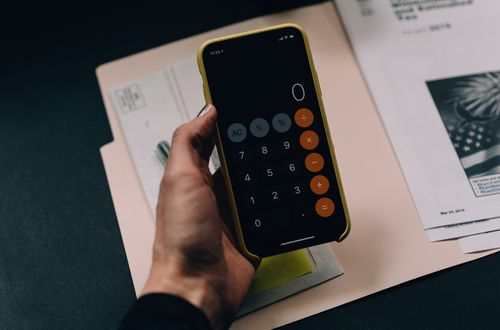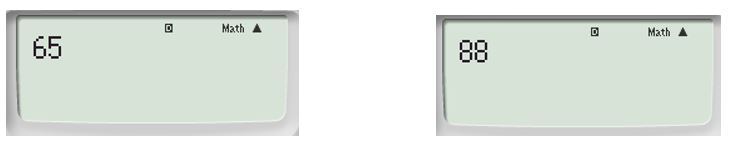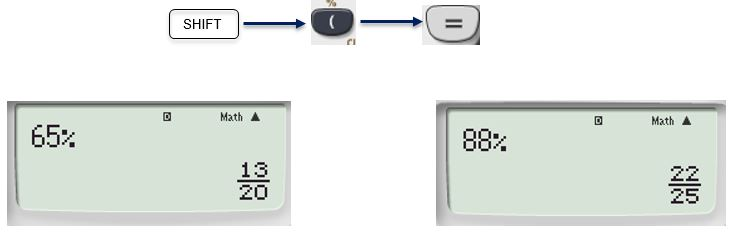# How to convert percentages into fractions using a calculator

Want to find out how to convert percentages into fractions using a calculator? We've provided a step-by-step guide of how to convert percentages into fractions using a calculator to teach you everything you need to know.## Step 1: Enter the value of the percentage that needs to be converted

For example, if we want to change 56%

## Step 2: Press the % button and the equal key

The result should show you the percentage in its fraction form.

Hence, we have 56%= 14/25 .

## Examples of how to convert percentages into fractions using a calculator

Q1) Use a scientific calculator to change the following to fractions. a. 65% b. 88%

Enter the values on the calculator first.Enter the % symbol then press the equal sign using the keys below as a guide.#### Join today

The fastest way to practice

Unlock our complete testing platform and improve faster that ever.